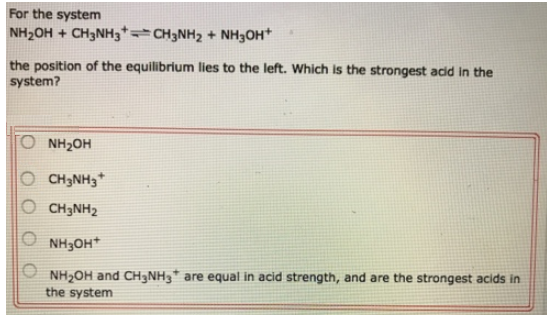# Problem: For the system NH2OH + CH3NH3+ ⇌ CH3NH2 + NH3OH+ the position of the equilibrium lies to the left. Which is the strongest acid in the system? (a) NH2OH(b)  CH3NH3+ (c) CH3NH2 (d) NH3OH+ (e) NH2OH and CH3NH3+ are equal in acid strength, and are the strongest acids in the system

###### FREE Expert Solution
92% (113 ratings)###### Problem Details

For the system

NH2OH + CH3NH3+ ⇌ CH3NH2 + NH3OH+

the position of the equilibrium lies to the left. Which is the strongest acid in the system?

(a) NH2OH

(b)  CH3NH3+

(c) CH3NH2

(d) NH3OH+

(e) NH2OH and CH3NH3+ are equal in acid strength, and are the strongest acids in the system